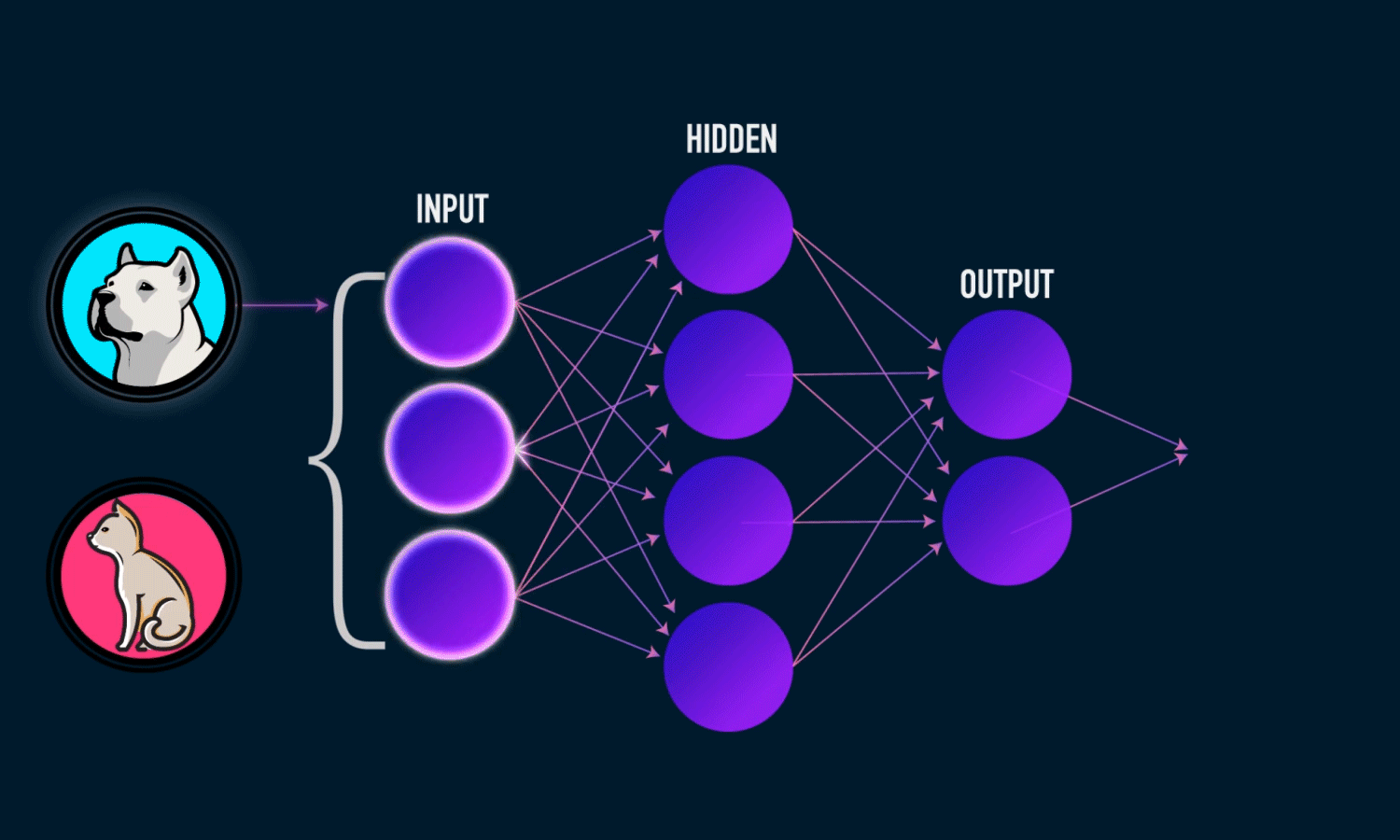# Deep Learning: The Learning Part

Source: Deep Learning on Medium

I tried introducing how learning happens on a Neural Network. Please leave a comment about it or about something else that you would want me to write next. Follow me and show some love clapping if you like it!

You started working with Deep Learning. You have a problem to solve. You go and choose a framework like Keras, TensorFlow, Pytorch. Then you build your network, give it examples and let it learns. Magically, it does really learn. But what does it mean to train a network? What is it performing under the hood?

The short answer for that is that the network learns because we update its internal weights to minimize the a loss function using the gradients calculated by a technique called Back Propagation.

Here I give an introduction on what this means. I divided in three steps: Forward Pass, Back Propagation and Weights Update.

### Forward Pass

The first step during training is to feed the network with an example. That means that we give the network some data and evaluate its output.

The image below shows how given a data, we can get a prediction. There is a small difference however between the normal prediction and how we do it during training. During training we calculate a loss function and not only the prediction itself.
This loss function is normally based on the output of the network. Taking for instance the network below for dogs and cats, we could tell the network that if it predicted wrongly, we would sum the value of 1 to a counter. If it predicted correctly, we would sum the value of 0. Then the resulting sum is the value of our loss function.

Normally other types of loss functions are used such as Cross Entropy. There are several different types and you can very well try your custom one.Getting a prediction on a network. Image from analyticsindiamag.com

After you calculate your loss function, you need a way to tune your network parameters so you minimize this loss. We do that by calculating gradients using Back Propagation and then updating these weights based on the gradients using Gradient Descent.

### Back Propagation

Back Propagation is the way that we calculate the gradients of the Network so we can update the weights.
I previously wrote an article about Convolutional Neural Networks and another one about Recurrent Neural Networks and how these architectures work. While they serve different applications — one is normally used with image and the other used with sequence data — the learning algorithm uses the same technique. They all use Back Propagation.

Back Propagation allows us to go layer by layer on the network and calculate its gradients with respect to the inputs and the weights. That means that you can start from the output layer where you have calculated your loss, then calculate the gradients for the layer right before the output one, then calculate the gradients for the layer before that one and so on. All that in a sequential manner.

This sequential calculation is possible because of the Chain Rule.

The image below shows how the gradients of the loss with respect to the inputs can be calculated using the gradient of the loss with respect to the output of the function using Chain Rule.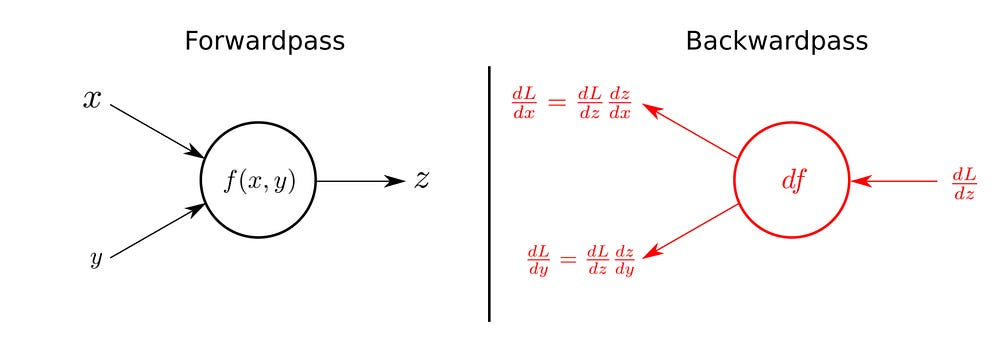Image showing how the gradients of the input of a function can be expressed using the gradient of the output. Image from kratzert

### How it is actually computed

We know from above that we need to update the weights based on the gradients of the network with relation to the error. However that starts to look complicated when we see that some networks have millions of weights to be updated. Using the traditional representation of Networks with nodes and arcs, where each arc is a single weight, makes it very hard to understand the calculations.

Computational Graphs are a way of organizing the thoughts while abstracting the number of weights in the network so we can calculate the gradients in a cleaner way.

Instead of representing the network as we traditionally do as a lot of little nodes and arcs, where each component could represent a single scalar number, we represent the network in terms of operations that the input goes through. That is independent on the number of inputs or the number of weights.

The picture below shows a network with a linear layer that is concatenated with a ReLU non-linear activation and then connected to a softmax output.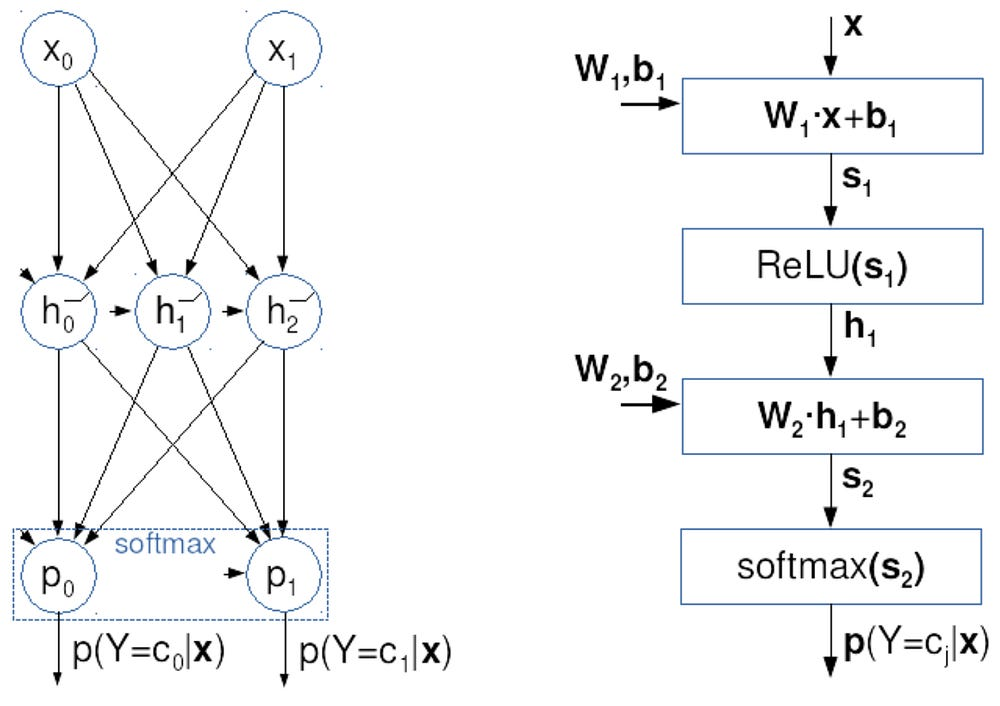Neuron Representation (Left) and Computational Graph Representation (Right) for the same network. Image from zemris.fer

Both left and right representations are equivalent. The distinction between them is that the left represents the exact number of inputs, weights and outputs. On the other hand, the same computational graph can be used even if there are thousands of inputs and weights. Therefore, it is a more general representation. In practice, computational graphs are used in all the libraries for Neural Networks such as Pytorch and TensorFlow.

The picture below shows how to compute the gradients using the computational graph from before using the chain rule.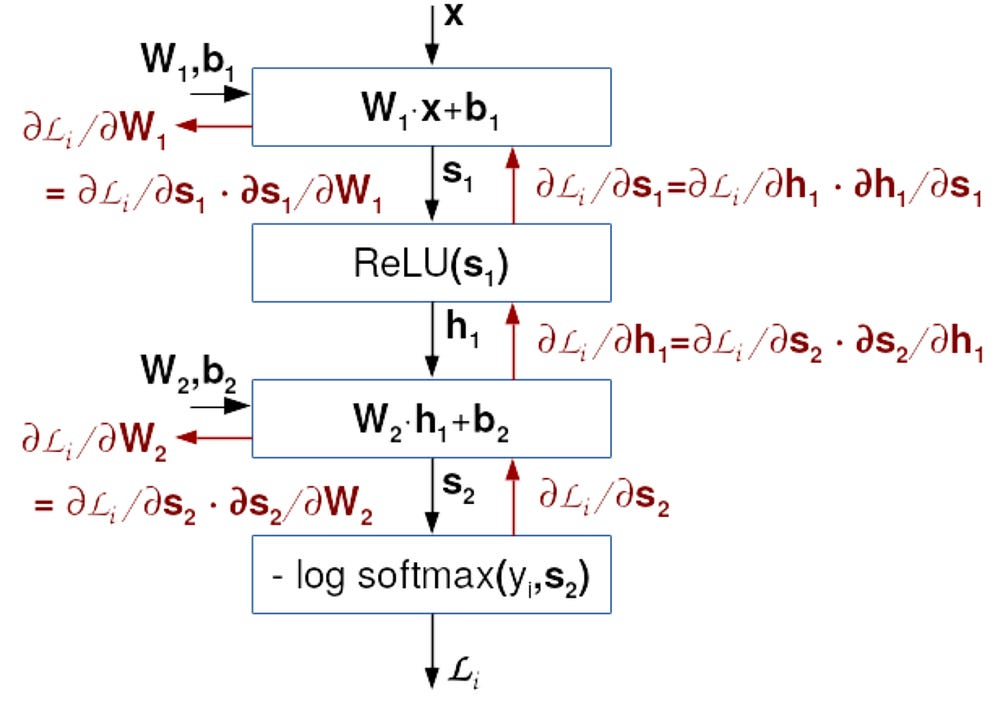How the gradients of each layer as calculated. Image from zemris.fer

Notice that the last layer was a modified to calculate the loss instead of only giving the prediction. The function in this case is the negative log likelihood, but we could have chosen something else.

If we look closely to the equalities in each of the layers, we can see that they are always expanded in two terms. One term is the derivative of the loss with respect to the output of the current layer and the other is the derivative of the current layer with respect to the variable we are interested in. That is all based on the Chain Rule.

That is the beauty of this algorithm. It makes possible to go layer by layer and calculate the derivatives.

### Updating the weights

We have the gradients, but how do we update the weights?

Updating is based on a technique called Gradient Descent. It tells us that we can minimize a function by updating its parameters using the gradients.

The equation below shows how to update the weights on our network.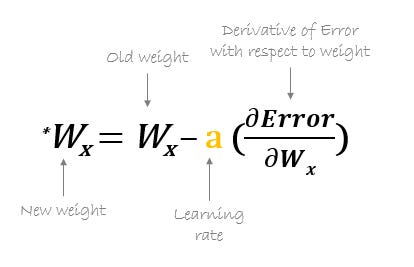Equation for weight update form hmkcode

Notice that there is a parameter called Learning Rate. That is the only parameter on the equation that is chosen by us. It determines how much we update the weights based on the gradients. If it is too big, we might never really minimize the loss because it can make it bounce from one side to the other. If it is too small, it might take too much time to reach a good value. There are several techniques used to choose good learning rates which we will not discuss here.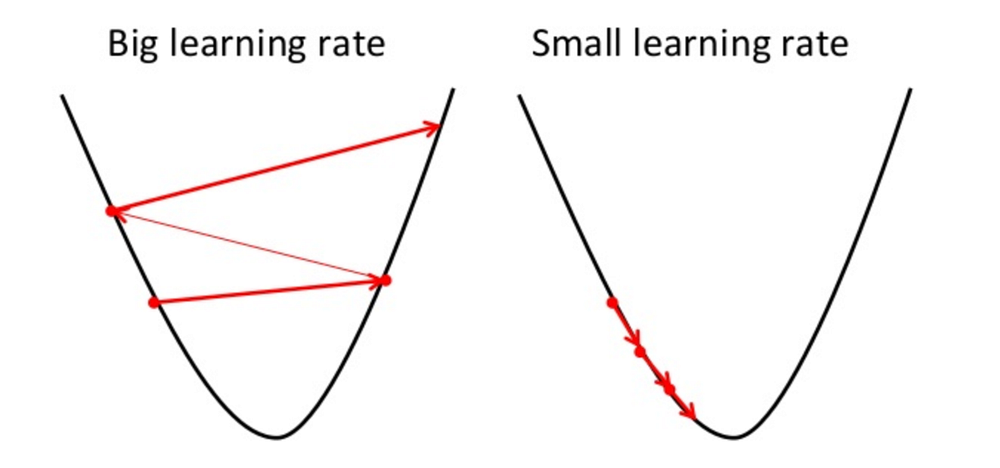Image from towardsdatascience.com showing the effects of too big and small learning rates.

We then need to keep performing the entire operation for several examples until the loss is good enough for us.

The entire process from forward pass, backward pass and weights update is in the picture below.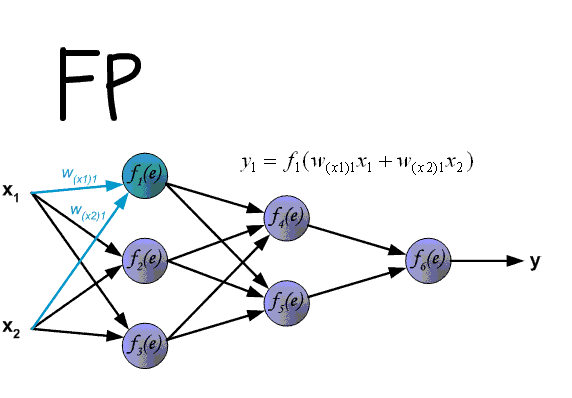Entire process of training a network from daniel-D

And that is how a network learns.